beginning of content:

Theorem 1: Suppose g is differentiable on an open interval containing x=c. If both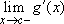and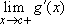exist, then the two limits are equal, and the common value is g'(c).

Proof: Let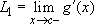and. By the Mean Value Theorem, for every positive h sufficiently small, there exists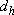satisfying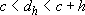such that: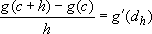.

Then: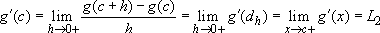.

Similarly, for every positive h sufficiently small, there exists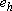satisfying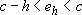such that: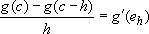.

Then: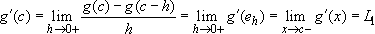. This shows that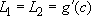.

Note: The same proof can be modified to show that if g is continuous at x=c and differentiable on both sides of x=c, and if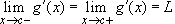, then g is differentiable at x=c with g'(c)=L.

Theorem 2: Suppose p and q are defined on an open interval containing x=c, and each are differentiable at x=c.

Let: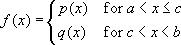Then f is differentiable at x=c if and only if p(c)=q(c) and p'(c)=q'(c).

Proof: We know that f'(c) exists if and only if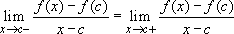.

We have that: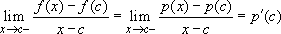.

Also: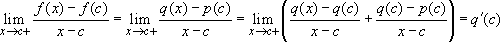if and only if p(c)=q(c). So f will be differentiable at x=c if and only if p(c)=q(c) and p'(c)=q'(c).

## 2003 AB6, part (c)

Suppose the function g is defined by: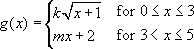where k and m are constants. If g is differentiable at x=3 what are the values of k and m?

### Method 1:

We are told that g is differentiable at x=3, and so g is certainly differentiable on the open interval (0,5).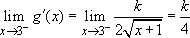and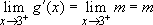.

So the two limits both exist and by Theorem 1 must be equal. Hence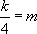. Since g is continuous at x=3, 2k=3m+2. This gives the two equations to solve for k and m.

### Method 2:

Let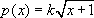and q(x)=mx+2. Both are differentiable at x=3. If g is differentiable at x=3, then Theorem 2 implies that p(3)=q(3) and p'(3)=q'(3). This yields the two same two equations as Method 1.

Either the note after Theorem 1 or Theorem 2 can be used to show that if we choose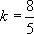and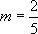, then we can prove that g is differentiable at x=3.

Jim Hartman teaches at the College of Wooster in Wooster, Ohio.

Larry Riddle is a professor of mathematics at Agnes Scott College in Decatur, Georgia.

## Authored by

• Jim Hartman
College of Wooster
Wooster, Ohio
• Larry Riddle
Agnes Scott College
Decatur, Georgia